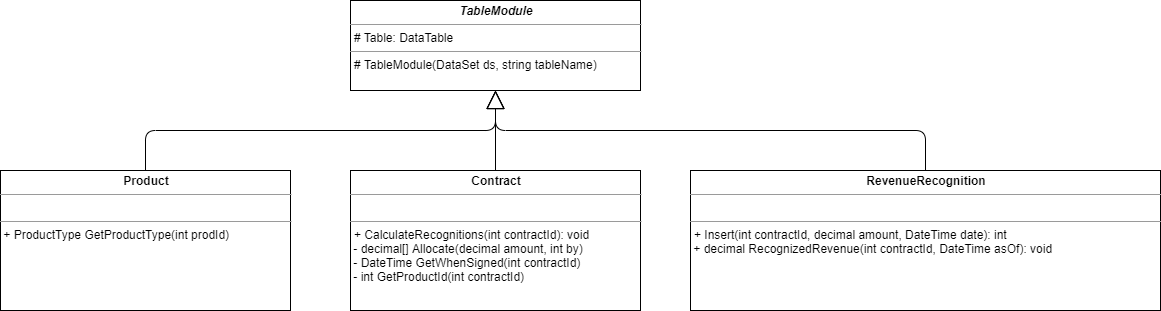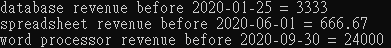0

# 1. What is Table Module

According to [PoEAA], this definition is "A single instance that handles the business logic for all rows in a database table or view."

A Table Module only processes one table/view/custom SQL query in a database. Its underlying data source pattern usually is Table Data Gateway. A approximate equality:

Table Module ≈ Table Data Gateway + Domain Logic

Table Module focuses on business logic and Table Data Gateway focuses on interactions with database (CRUD).

## 1.1 When to use it

If the business layer is based on the structure of database table, Table Module is a better choice. When business logic becomes more complex, it's better to choose Domain Logic as business layer.

# 2. Pattern Practice: The Revenue Recognition Problem

This problem is introduced in the previous article [PoEAA] Domain Logic Pattern - Transaction Script. This article uses Table Module to build the domain layer.

Define the table module classes for the revenue recognition, product and contract as the following figure:Figure 1: Class diagram of Revenue Recognition example

## 2.1 Implementation by C#

This pattern is implemented by C#. Because the section Chapter 9 Domain Logic Pattern - Table Module of PoEAA didn't implement with database integration, this implementation don't contain database example.

### 2.1.1 Table Module base class

.NET's DataSet is a type of a in-memory database structure and provides basic CRUD functions. And DataTable is a table structure and is contained in a DataSet, so every Table Module contains a DataTable object.

``````abstract class TableModule
{
protected DataTable Table;

protected TableModule(DataSet ds, string tableName)
{
Table = ds.Tables[tableName];
}
}
``````

### 2.1.2 Product Class

For Product class, it contains a DataTable named "Products" and a function that returns ProductType by product id:

``````public enum ProductType
{
W,
S,
D
};

class Product : TableModule
{
public Product(DataSet tableDataSet) : base(tableDataSet, "Products")
{
}

public DataRow this[int key]
{
get
{
string filter = \$"Id = {key}";
return Table.Select(filter);
}
}

public ProductType GetProductType(int prodId)
{
string typeCode = (string) this[prodId]["Type"];
return (ProductType) Enum.Parse(typeof(ProductType), typeCode);
}
}
``````

### 2.1.3 Contract Class & RevenueRecognition Class

For Contract class, it contains a DataTable named "Contracts" and a function that calculates the recognized revenues. It associates a RevenueRecognition table module and inserts the revenue to RevenueRecognition.

For RevenueRecognition class, it contains a DataTable named "RevenueRecognitions". One function that inserts revenue to DataTable. The second function that returns revenue by contract id and a date.

``````class Contract : TableModule
{
public Contract(DataSet ds) : base(ds, "Contracts")
{
}

public DataRow this[int key]
{
get
{
string filter = \$"Id = {key}";
return Table.Select(filter);
}
}

public void CalculateRecognitions(int contractId)
{
DataRow contractRow = this[contractId];
decimal amount = (decimal) contractRow["Revenue"];
RevenueRecognition rr = new RevenueRecognition(Table.DataSet);
Product prod = new Product(Table.DataSet);
int prodId = GetProductId(contractId);

var productType = prod.GetProductType(prodId);

if (productType == ProductType.W)
{
rr.Insert(contractId, amount, GetWhenSigned(contractId));
}
else if (productType == ProductType.S)
{
DateTime signedDate = GetWhenSigned(contractId);
decimal[] allocation = Allocate(amount, 3);
rr.Insert(contractId, allocation, signedDate);
}
else if (productType == ProductType.D)
{
DateTime signedDate = GetWhenSigned(contractId);
decimal[] allocation = Allocate(amount, 3);
rr.Insert(contractId, allocation, signedDate);
}
}

private decimal[] Allocate(decimal amount, int by)
{
decimal lowResult = amount / by;
lowResult = decimal.Round(lowResult, 2);
decimal highReult = lowResult + 0.01m;
decimal[] results = new decimal[by];
int remainder = (int) amount % by;
for (int i = 0; i < remainder; ++i)
{
results[i] = highReult;
}

for (int i = remainder; i < by; ++i)
{
results[i] = lowResult;
}

return results;
}

private DateTime GetWhenSigned(int contractId)
{
return (DateTime)this[contractId]["DateSigned"];
}

private int GetProductId(int contractId)
{
return (int)this[contractId]["Product"];
}
}

class RevenueRecognition : TableModule
{
private static int _id = 1;
private static readonly object IdLock = new object();
public RevenueRecognition(DataSet ds) : base(ds, "RevenueRecognitions")
{
}

public int Insert(int contractId, decimal amount, DateTime date)
{
DataRow newRow = Table.NewRow();
int id = GetNextId();
newRow["Id"] = id;
newRow["Contract"] = contractId;
newRow["Amount"] = amount;
newRow["RecognizedOn"] = date;

return id;
}

public decimal RecognizedRevenue(int contractId, DateTime asOf)
{
string filter = \$"Contract = {contractId} AND RecognizedOn <= #{asOf:d}#";
DataRow[] rows = Table.Select(filter);
decimal result = 0m;
foreach(DataRow row in rows)
{
result += (decimal) row["Amount"];
}

return result;
}

private static int GetNextId()
{
lock (IdLock)
{
return _id++;
}
}
}
``````

### 2.1.4 Demo

Create a console program and create 3 Products and 3 Contracts to calculate the revenue recognitions for the 3 products.

As the following code:

``````// mock Result Set
DataSet ds = new DataSet();

DataTable productTable = new DataTable("Products");

DataTable contractTable = new DataTable("Contracts");
contractTable.Rows.Add(2, 2, 1000, new DateTime(2020, 3, 15));
contractTable.Rows.Add(3, 3, 24000, new DateTime(2020, 7, 25));

DataTable revenueRecognitionsTable = new DataTable("RevenueRecognitions");

// calculate recognized revenues
Contract contract = new Contract(ds);

// database product
contract.CalculateRecognitions(1);
var databaseRevenue = new RevenueRecognition(ds).RecognizedRevenue(1, new DateTime(2020, 1, 25));
Console.WriteLine(\$"database revenue before 2020-01-25 = {databaseRevenue}");

contract.CalculateRecognitions(2);
var spreadsheetRevenue = new RevenueRecognition(ds).RecognizedRevenue(2, new DateTime(2020, 6, 1));

// word processor product
contract.CalculateRecognitions(3);
var wordProcessorRevenue = new RevenueRecognition(ds).RecognizedRevenue(3, new DateTime(2020, 9, 30));
Console.WriteLine(\$"word processor revenue before 2020-09-30 = {wordProcessorRevenue}");
``````

The console shows:This result is the same as the Transaction Script example.

# 3. Conclusions

"Table Module" is good at processing every table's logic. For .NET solution, DataSet/DataTable have provide complete functions to use this pattern. But when the domain layer is more complex, Domain Model pattern is the better choice.

The above sample code is uploaded to this Github Repository.

For next article I will write Service Layer pattern according to Chapter 9 Domain Logic Pattern - Service Layer of PoEAA.

# 4. References

Patterns of Enterprise Application Architecture Book(Amazon)